Checkout JEE MAINS 2022 Question Paper Analysis : Checkout JEE MAINS 2022 Question Paper Analysis :

# Circles in Maths

In Maths or Geometry, a circle is a special kind of ellipse in which the eccentricity is zero and the two foci are coincident. A circle is also termed as the locus of the points drawn at an equidistant from the centre. The distance from the centre of the circle to the outer line is its radius. Diameter is the line which divides the circle into two equal parts and is also equal to twice of the radius.

A circle is a basic 2D shape which is measured in terms of its radius. The circles divide the plane into two regions such as interior and exterior region. It is similar to the type of line segment. Imagine that the line segment is bent around till its ends join. Arrange the loop until it is precisely circular.

The circle is a two-dimensional figure, which has its area and perimeter. The perimeter of the circle is also called the circumference, which is the distance around the circle. The area of the circle is the region bounded by it in a 2D plane. Let us discuss here circle definition, formulas, important terms with examples in detail.

## Circle Definition

A circle is a closed two-dimensional figure in which the set of all the points in the plane is equidistant from a given point called “centre”. Every line that passes through the circle forms the line of reflection symmetry. Also, it has rotational symmetry around the centre for every angle. The circle formula in the plane is given as:

(x-h)2 + (y-k)2 = r2

where (x,y) are the coordinate points
(h,k) is the coordinate of the centre of a circle
and r is the radius of a circle.

### Circle Shaped Objects

There are many objects we have seen in the real world that are circular in shape. Some of the examples are:

• Ring
• CD/Disc
• Bangles
• Coins
• Wheels
• Button
• Dartboard
• Hula hoop

We can observe many such examples in our day to day life.

## How to Draw a Circle?

In maths projects for class 10 on circles, the construction of a circle, all the properties and terminologies are explained in detail. To understand what circles are in simple terms, go through circles for class 10, and also try the following exercise –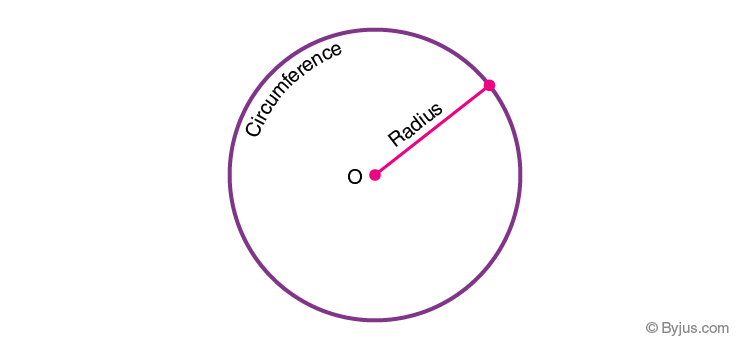1. Take an empty sheet of paper and mark a single point on the sheet, somewhere in the middle of the sheet, and name it to point O.
2. Select a random length for radius, for example, 3 cm.
3. Using a ruler, keep the reference zero mark on point O and randomly mark 3 cm away from point O in all the direction.
4. Mark as many points as you want away from point O, but all of them should be exactly 3 cm away from point O.

If you’ve selected sufficient points, you may notice that the shape is starting to resemble a circle and this is exactly what the definition of a circle is.

## Parts of Circle

A circle has different parts based on the positions and their properties. The different parts of a circle are explained below in detail.

• Annulus-The region bounded by two concentric circles. It is basically a ring-shaped object. See the figure below.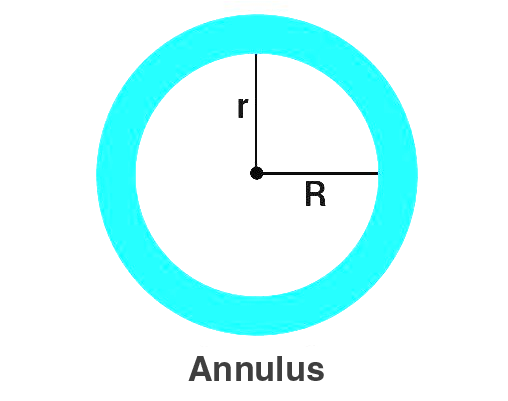• Arc – It is basically the connected curve of a circle.
• Sector – A region bounded by two radii and an arc.
• Segment- A region bounded by a chord and an arc lying between the chord’s endpoints. It is to be noted that segments do not contain the centre.

See the figure below explaining the arc, sector and segment of a circle.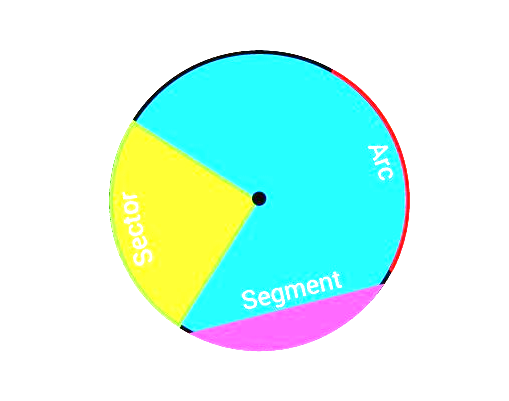• Centre – It is the midpoint of a circle.
• Chord- A line segment whose endpoints lie on the circle.
• Diameter- A line segment having both the endpoints on the circle and is the largest chord of the circle.
• Radius- A line segment connecting the centre of a circle to any point on the circle itself.
• Secant- A straight line cutting the circle at two points. It is also called an extended chord.
• Tangent- A coplanar straight line touching the circle at a single point.

See the figure below-representing the centre, chord, diameter, radius, secant and tangent of a circle.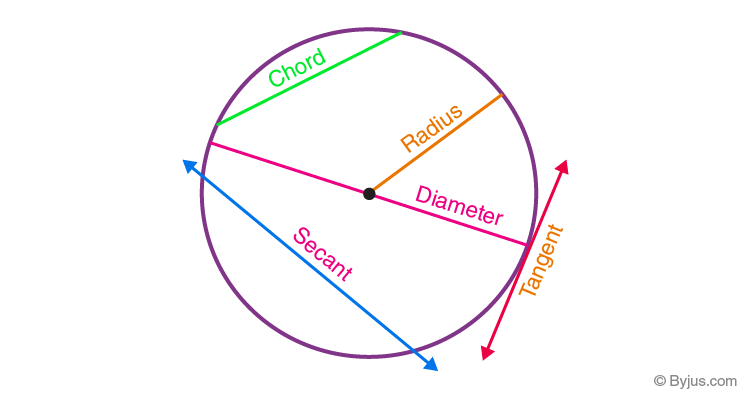A line segment connecting the centre of a circle to any point on the circle itself “. The radius of the circle is denoted by “R” or “r”.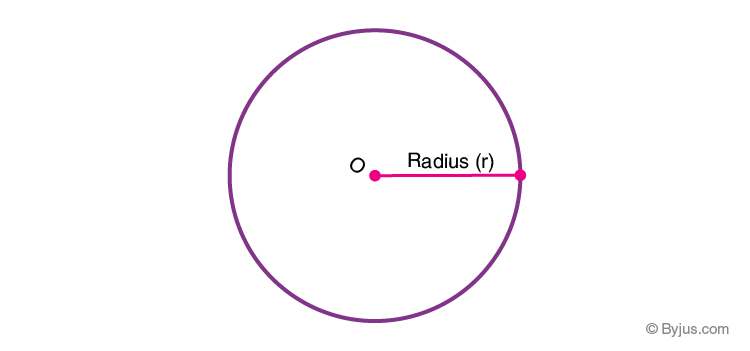### Diameter (d) of Circle

A line segment having both the endpoints on the circle. It is twice the length of radius i.e. d = 2rFrom the diameter, the radius of the circle formula is obtained as r= d/2.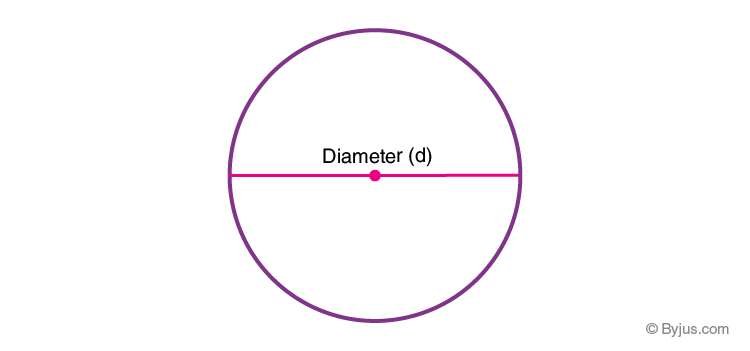## Circle Formulas

We know that a circle is a two-dimensional curve-shaped figure, and the two different parameters used to measure the circle are:

• Area of  circle
• Circumference of a circle

Let us discuss here the general formulas for area and perimeter/circumference of a circle.

### Area and Circumference of a Circle

 Circumference (C) The circumference of a circle is defined as the distance around the circle. The word ‘perimeter’ is also sometimes used, although this usually refers to the distance around polygons, figures made up of the straight line segment. A circle circumference formula is given by   C = πd = 2 π r Where, π = 3.1415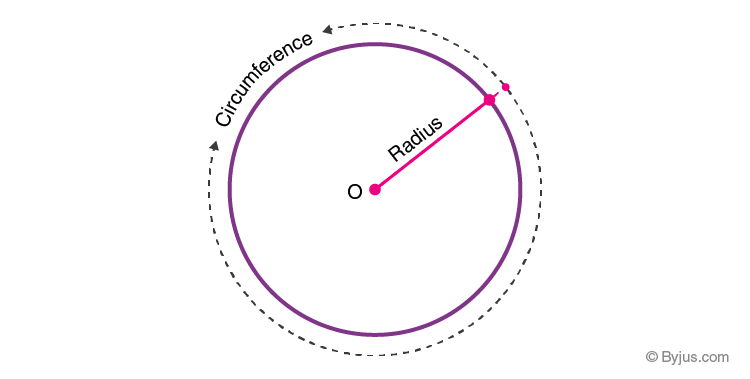Area (A) Area of a circle is the amount of space occupied by the circle. The circle formula to find the area is given by Area of a circle = πr2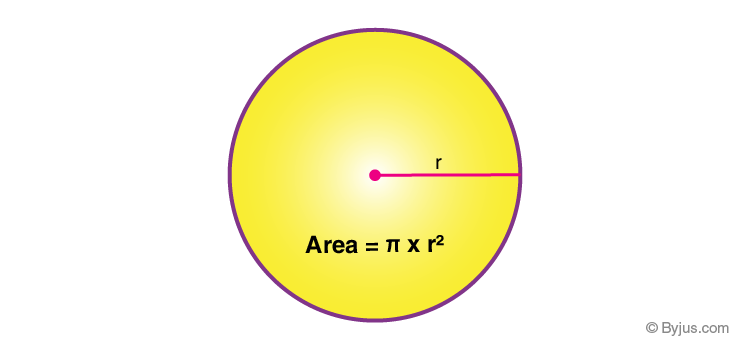### Circle Area Proof

We know that Area is the space occupied by the circle.

Consider a concentric circle having an external circle radius to be ‘r.’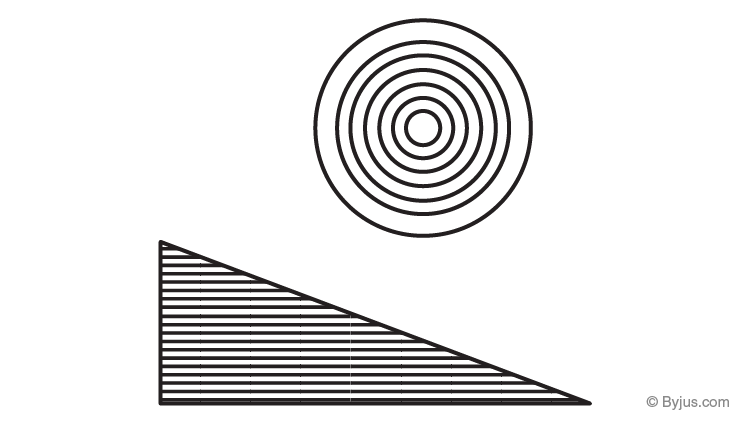Open all the concentric circles to form a right-angled triangle.

The outer circle would form a line having length 2πr forming the base.

The height would be ‘r’

Therefore the area of the right-angled triangle formed would be equal to the area of a circle.

Area of a circle = Area of triangle = (1/2) ×b ×h

= (1/2) × 2π r  × r

Therefore, Area of a circle = πr2

### Properties of Circles

The important basic properties of circles are as follows:

• The outer line of a circle is at equidistant from the centre.
• The diameter of the circle divides it into two equal parts.
• Circles which have equal radii are congruent to each other.
• Circles which are different in size or having different radii are similar.
• The diameter of the circle is the largest chord and is double the radius.

## Video Lessons on Circles

### Introduction to Circles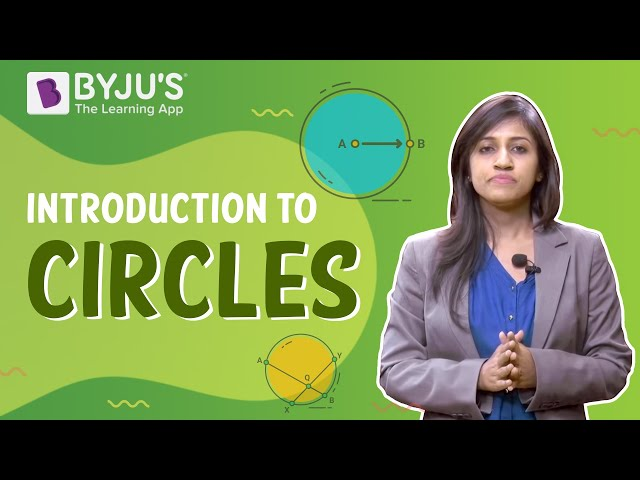### Parts of a Circle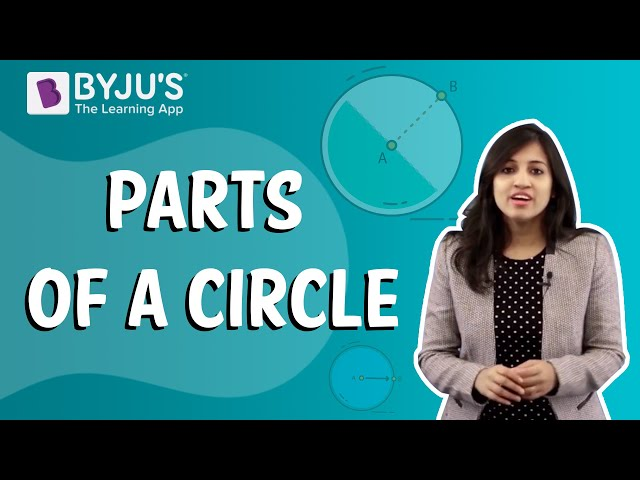### Area of a Circle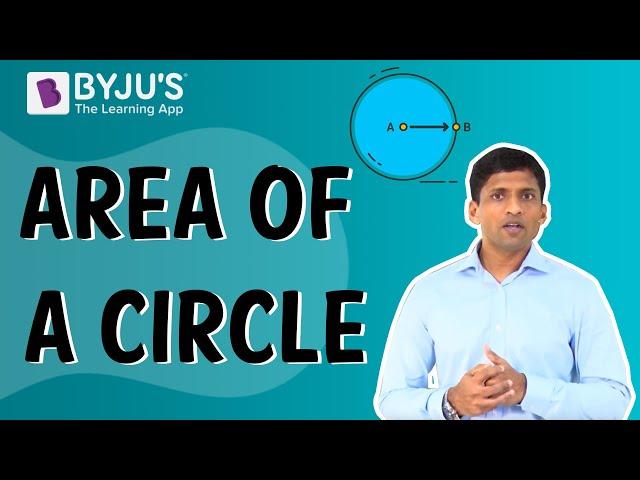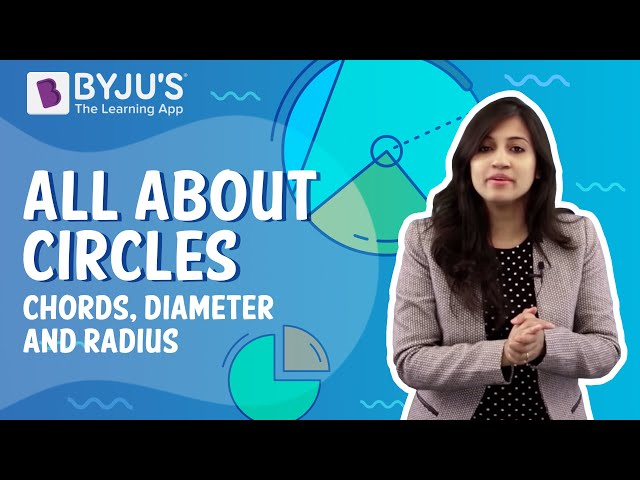## Solved Examples

Example 1:

Find the area and the circumference of a circle whose radius is 10 cm. (Take the value of π = 3.14)

Solution:

Area =π r

= 3.14  × 102

A= 314 cm2

Circumference, C = 2πr

C= 2 ×3.14×  10

Circumference= 62.8 cm

Example 2:

Find the area of a circle whose circumference is 31.4 cm.

Solution:

Given:

Circumference = 31.4 cm

To find the area of a circle, we need to find the radius.

From the circumference, the radius can be calculated:

2 π r = 31.4

(2)(3.14)r = 31.4

r = 31.4 /(2)(3.14)

r=10/2

r= 5

Therefore, the radius of the circle is 5 cm.

The area of a circle is πr2 square units

Now, substitute the radius value in the area of a circle formula, we get

A = π(5)2

A = 3.14 x 25

A =  78.5 cm2

Therefore, the area of a circle is 78.5 cm2.

### Practice Problems

Solve the following circle problems given below:

1. Find the area of a circle whose radius is 7 cm
2. Find the circumference of a circle whose radius is 9 cm
3. The area of a circle is 176 cm2. Find its radius

## Frequently Asked Questions on Circles

### What is called a circle?

A circle is a closed two-dimensional curve shaped figure, where all the points on the surface of the circle are equidistant from the centre point.

### What are the different parts of a circle?

The different parts of a circle are radius, diameter, chord, tangent, arc, centre, secant, sector.

### Write down the circle formulas.

If “r” is the radius of the circle, then the formula for the area and the circumference of a circle are:
Circumference of a Circle = 2πr units
Area of a circle = πr2 square units.

### Define radius and diameter of a circle.

The radius of a circle is the line segment that connects the centre point and the circle surface. The diameter is considered as the longest chord of a circle which is twice the radius.

### Define chord

The chord of a circle is defined as the straight line segment whose both endpoints touch the surface of a circle. The longest chord of a circle is a diameter.

Stay tuned with BYJU’S – The Learning App to learn more maths-related articles and also watch engaging videos to learn with ease.